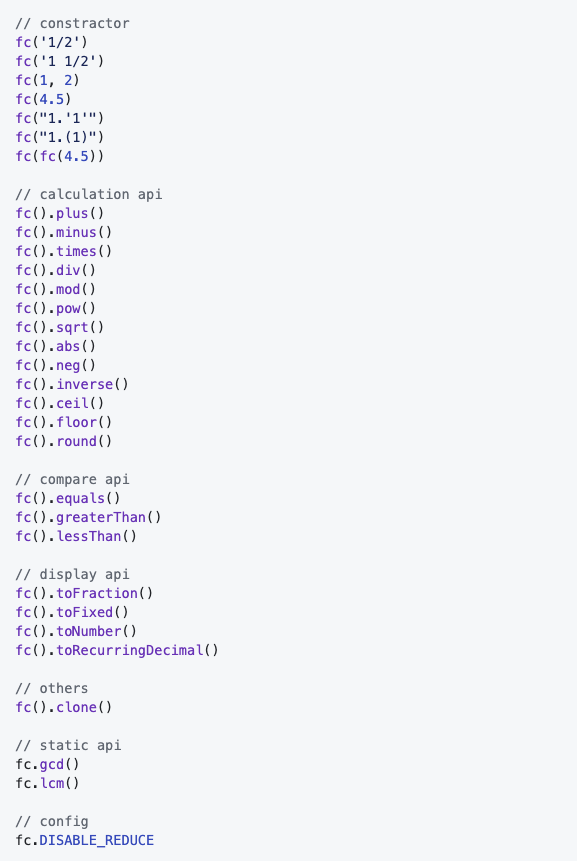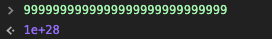# 学以致用：手把手教你撸一个工具库并打包发布，顺便解决JS小数计算精度问题

2019/04/10 10:10

Math.sqrt(Math.pow(((1/3+3.5)*2/9-27/109)/(889/654),4));   //  0.1975308641975308


fc('1/3')
.plus(3.5)
.times('2/9')
.minus('27/109')
.div('889/654')
.pow(4)
.sqrt()
.toFraction();     // 输出： 16/81


fc('16/81').toRecurringDecimal(); // "0.(197530864)"


fc('0.(197530864)').toFraction(); // 16/81


0.1 + 0.2;     // 0.30000000000000004
fc(0.1).plus(0.2).toNumber(); // 0.3


npm install fraction-calculator --save


GitHub地址： https://github.com/dennis-jiang/fraction-calculator

GitHub上有详细的使用说明，本文接下来的篇幅会详细讲解怎么实现功能和打包发布。

## 功能实现

### API一览1. 构造器
2. 计算API
3. 比较API
4. 输出显示API
5. 静态API
6. 其他API
7. 配置

### 构造器

1. 不用new就可以直接使用
2. 支持字符串的分数，包括有整数部分或者没有整数部分
3. 支持数字
4. 支持循环小数
5. 支持另一个实例
6. 支持两个数字参数

#### 从去掉new开始构建架构

= 10.714285714285714285714285714285

-> 0.10(714285) // 前面乘了两个10，小数点左移两位function getDecimalsFromFraction(numerator, denominator) {
// make sure numerator is less than denominator
const modObj = {};
const quotientArray = [];

let mod;
let index = 0;

while (true) {
mod = numerator % denominator;

if (mod === 0) {
return quotientArray.join('');
}

let existIndex = modObj[mod];
if (existIndex >= 0) {
let quotientLength = quotientArray.length;
quotientArray.splice(existIndex, 0, '(');
quotientArray.splice(quotientLength + 1, 0, ')');

return quotientArray.join('');
}

modObj[mod] = index;
index++;
numerator = mod * 10;

let quotient = parseInt(numerator / denominator);
quotientArray.push(quotient);

if (index >= 3000) {
// Recurring part can be very long, we only handle first 3000 numbers
return quotientArray.join('');
}
}
}


### 静态API

fc有两个静态API，gcdlcm，这其实就是我们前面计算用到的最大公约数和最小公倍数，既然都写出来了，为啥不顺便暴露给用户用呢？

## 单元测试

describe('FractionCalculator instance', () => {
it('can support integer', () => {
const instance = fc(4);

expect(instance.fraction).toEqual({
numerator: 4,
denominator: 1,
});
});
});


## 打包发布

1. 流行的ES6，node.js要支持
2. 浏览器要支持
3. 老的浏览器，比如IE，尽量支持

{
...
library: 'fc',                 // 库名字，也是script引入时挂载到window的对象名字
libraryTarget: 'umd',          // 支持的引入方式，umd是包括ES6, node, 浏览器，AMD等
libraryExport: 'default',      // 默认导出的路径，我用export default导出的就写'default'
...
}


{
...
"useBuiltIns": "usage"     // 关键就是这个配置，这个只会添加用到了的polyfill
...
}


1. fraction-calculator.js没有压缩，没有polyfill的版本，供ES6和node使用，package.json里面的main也指向的这个包，这样用户npm安装之后，import或者require的就是这个文件
2. fraction-calculator.min.js压缩版的fraction-calculator.js，供高级浏览器使用，比如火狐，Chrome，高级浏览器自己支持ES6，就不用polyfill了，这个文件体积也最小，只有7kb
3. fraction-calculator.polyfill.min.js加了polyfill的fraction-calculator.min.js，体积会稍微大一点，供IE之类的使用。

## 总结

fc项目GitHub地址： https://github.com/dennis-jiang/fraction-calculator

0
0 收藏

### 作者的其它热门文章0 评论
0 收藏
0